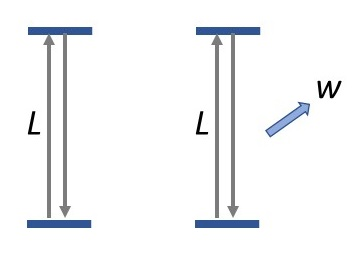# Temporo-spatial light clock

This post builds on the post about the Michelson-Morley experiment here.

One “Derivation of time dilation” (e.g., here) uses a light clock, pictured below:The illustration on the left shows a light clock at rest, with a light beam reflecting back and forth between two mirrors. The distance of travel is set at the beginning with the separation of the mirrors. It is like a race: one lap is a round-trip between the two mirrors. So the independent variable is distance, and the dependent variable is time. This is key to properly understanding the experiment.

In the stationary frame the round trip longitudinal distance between the two mirrors is 2L, the pace is 1/c, and the time of one cycle is T = 2L/c.

The illustration on the right shows an observer moving with lenticity wt transversely to the light clock. In this case there are two components of motion: the longitudinal axis and the transverse axis. These components are independent of one another since they are in different dimensions. The transverse axis is the same as the stationary case above: the total distance is 2L and the total time is 2L/c. The mean pace is 2T/(2cT) = 1/c.

The longitudinal axis is simply the Galilean transform: t′ = t + wx since motion in different dimensions is independent. If the light clocks coincide at t = 0, this is t′ = wx, which is what was given.

Thus the distance, time, and mean speed are the same for both observers and independent of their relative velocity, which is what the Michelson-Morley experiment found, though didn’t expect.